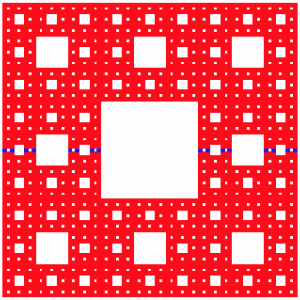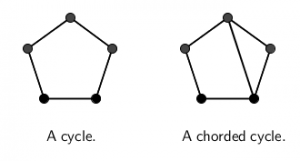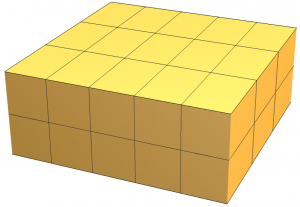Descriptions of the three research groups are given below.

Geometry: Short Paths in Fractals
Professors Ethan Berkove and Derek Smith

The Cantor set, $$C$$, is a well-known fractal that can be built in stages.  Start with the closed unit interval $$[0,1]$$, which we call the level-0 approximation to $$C$$.  Remove the open middle third interval $$(1/3,2/3)$$; we call the union of the remaining two closed intervals of length 1/3 the level-1 approximation to $$C$$.  Remove the two middle open thirds of those intervals to get the level-2 approximation, a union of four closed intervals of length 1/9.  Repeat this process indefinitely; the limiting set is the full Cantor set, $$C$$, a 1-dimensional fractal that contains no intervals.

The Sierpinski carpet, $$S$$, is a 2-dimensional version of the Cantor set, built in a similar fashion.  Starting with a unit square, subdivide it into nine squares of side length 1/3 and remove the open center square to get the level-1 approximation to $$S$$, consisting of eight closed squares of side length 1/3.  Repeat the process in a similar way to the Cantor set construction, and you soon get the picture below, which shows a level-4 approximation to $$S$$ in red, containing a copy of the level-4 approximation to $$C$$ in blue.  Repeat the process indefinitely, and you get the full Sierpinski carpet, $$S$$.Unlike with the Cantor set, it is possible to travel between any two points in the Sierpinski carpet while staying within the fractal.  In fact, there are infinitely many different paths from one point to another, including paths that don’t always travel horizontally or vertically.  But what’s a geodesic, a shortest path between two points?  In this project, we will research several notions of shortest path in the Sierpinski carpet and its generalizations, including higher-dimensional fractals like the Menger sponge.

Applicants for the project should have completed multivariable calculus and a proof-based math class by the start of the summer program.

Graph Theory: Conditions for Chorded $$(k,m)$$-Pancyclicity
Professor Megan Cream

A graph $$G$$ is called pancyclic if it contains a cycle of every possible length from 3 up to the order of the graph. Further, we say that $$G$$ is vertex-pancyclic if every vertex of $$G$$ is contained in a cycle of every possible length. The property of vertex-pancyclicity can be further generalized to $$(k, m)$$-pancyclicity, which requires every set of $$k$$ vertices in $$G$$ to be contained in a cycle of length $$i$$ for each $$i\in\{m, m+1, m+2, \dots, n\}$$, where $$n$$ is the order of the graph. The relationships between these cycle properties and bounds on degree-type conditions are well-studied. In 2004 Faudree, Gould, Jacobson, and Lesniak showed that a degree-sum bound ensures a particular kind of $$(k, m)$$-pancyclicity. Another method used to guarantee such cycle properties in graphs is forbidding a subgraph or a family of subgraphs as induced subgraphs. Last year, Crane characterized pairs of forbidden subgraphs that guarantee $$(k,m)$$-pancyclicity in graphs.

In this project, we will stretch the property of $$(k, m)$$-pancyclicity to chorded cycles — that is, cycles with at least one edge between two vertices that are not adjacent on the cycle itself (an example is shown below). Thus a graph $$G$$ of order $$n$$ is chorded $$(k,m)$$-pancyclic if every set of $$k$$ vertices in $$G$$ is contained in a chorded cycle of length $$i$$ for each $$i\in \{m, m+1, m+2, \ldots, n\}$$. Our goal for this project will be to determine conditions that guarantee this newly-defined cycle property. These conditions may range from degree and degree-sum conditions to forbidden subgraphs, as well as other conditions we encounter or define along the way.Interested applicants must have familiarity and experience with writing mathematical proofs, through the completion of an introduction to proofs course or any proof-based mathematics course by the start of the summer program. A course in discrete mathematics, combinatorics, and/or graph theory may be beneficial, but is not required.

Recreational Mathematics: Exploring Sophisticated Mathematics through Seemingly Simple Problems
Professors Gary Gordon and Brittany Shelton

Many deep and surprising mathematical ideas can be found in recreational problems. At the beginning of the program, we will consider several open-ended problems with an emphasis on combinatorics and/or discrete geometry. As the summer progresses, we will narrow our focus and deepen our study of these problems.

As an example of the kind of problem we may work on, consider a 3-dimensional rectangular box with dimensions $$a\times b \times c$$, where $$a$$, $$b$$, and $$c$$ are positive integers. A game is played as follows. The box is filled with $$a\times b\times c$$ unit cubes, and each unit cube has unique $$(x,y,z)$$-coordinates in the box. You then select a cube with coordinates $$(r,s,t)$$ and you remove it; this also removes all the unit cubes in the three lines through this cube parallel to the edges of the box. For instance, if you choose $$(1,2,3),$$ then all cubes with coordinates $$(x,2,3), (1,y,3)$$ and $$(1,2,z)$$ will be removed. (The remaining cubes stay in place – there is no gravity in this problem.)  You continue to remove unit cubes in this fashion.There are several questions to explore:

• What is the minimum number of steps needed to remove all the cubes in the box?
• If there are two players who alternate turns, with the person who removes the last cube the loser, who will win? (This will obviously depend on the dimensions of the box.)
• What if the winner is the person who removes the final cube?
• What if there are more than two players?
• What happens if the cubes shift, using gravity, after each move?
• What happens in other dimensions?

And so on.

This sample project is based on Problem 327 in the Sept. 2015 Math Horizons problem solving column, The Playground. See the Feb. 2016 and Sept. 2016 issues of Math Horizons for an argument that finds the shortest number of steps needed to remove all the cubes in an initial configuration of $$5 \times 13 \times 31 = 2015$$ cubes.

Applicants should have completed a discrete mathematics course or an introduction to proof course by the start of the summer program.